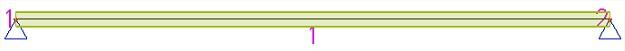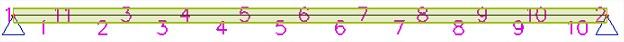Error modal analysis: "Number of the nonzero numbers in the mass vector should be at least twice as big as it is".

If the following error message appears during a modal analysis ...

... it means that a fewer amount of eigenmodes than indicated in the Solver Setup can be calculated.

By default the first 4 eigenfrequencies are calculated by SCIA Engineer:

Let’s take a look at the example of a simply supported beam, for which this message might appear at the start of the modal analysis.The explication is as follows: by default there is only one finite mesh element per beam. The consequence is that the mass of the beam will be completely divided to the two end nodes, which are both rigidly supported for displacements in each direction. This means that in the calculation model the mass cannot move which results in zero degrees of freedom for the mass movement ... so zero eigenmodes can be calculated.

A solution is to augment the number of mesh elements per 1D member, via the Setup menu > Mesh:

Now the mass can be divided to a lot more mesh nodes (without displacement restrictions), which results in a lot more degrees of freedom for the mass movement.The modal analysis of the first 4 eigenfrequencies can now be finished without any problems.